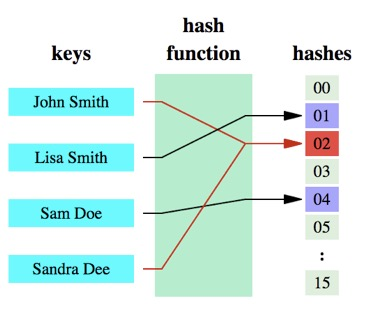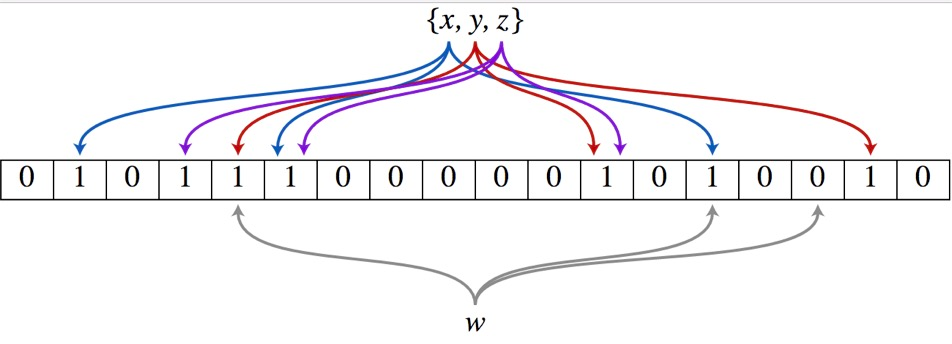# 布隆过滤器(bloom filter)介绍以及php和redis实现布隆过滤器实现方法

## 引言## 布隆过滤器原理## 数学推导

$$\frac{1}{m}$$

$$p(1)=\frac{1}{m}$$

$$p(0)=1-\frac{1}{m}$$

$$p(0)=(1-\frac{1}{m})^{nk}$$

$$p(误判)=1-(1-\frac{1}{m})^{nk}$$

$$p(误判)=(1-(1-\frac{1}{m})^{nk})^k$$

$$(1-\frac{1}{m})^{nk}$$

$$\lim\limits _{x\to\infty} (1+\frac{1}{x})^x = e$$

$$p(误判) = (1-(e)^{-nk/m})^k$$

$$p^{’} \frac{1}{p} = ln(1-e^{tk}) + \frac{ k lne^{t} (-e^{tk}) } {1-e^{tk}}$$

$$(1-e^{tk}) ln(1-e^{tk}) = e^{tk}lne^{tk}$$

$$(1/2)^{ln2 \frac{m}{n}}$$，约等于$$0.6185^{m/n}$$

 m/n k k=1 k=2 k=3 k=4 k=5 k=6 k=7 k=8 2 1.39 0.393 0.400 3 2.08 0.283 0.237 0.253 4 2.77 0.221 0.155 0.147 0.160 5 3.46 0.181 0.109 0.092 0.092 0.101 6 4.16 0.154 0.0804 0.0609 0.0561 0.0578 0.0638 7 4.85 0.133 0.0618 0.0423 0.0359 0.0347 0.0364 8 5.55 0.118 0.0489 0.0306 0.024 0.0217 0.0216 0.0229 9 6.24 0.105 0.0397 0.0228 0.0166 0.0141 0.0133 0.0135 0.0145 10 6.93 0.0952 0.0329 0.0174 0.0118 0.00943 0.00844 0.00819 0.00846 11 7.62 0.0869 0.0276 0.0136 0.00864 0.0065 0.00552 0.00513 0.00509 12 8.32 0.08 0.0236 0.0108 0.00646 0.00459 0.00371 0.00329 0.00314 13 9.01 0.074 0.0203 0.00875 0.00492 0.00332 0.00255 0.00217 0.00199 14 9.7 0.0689 0.0177 0.00718 0.00381 0.00244 0.00179 0.00146 0.00129 15 10.4 0.0645 0.0156 0.00596 0.003 0.00183 0.00128 0.001 0.000852 16 11.1 0.0606 0.0138 0.005 0.00239 0.00139 0.000935 0.000702 0.000574 17 11.8 0.0571 0.0123 0.00423 0.00193 0.00107 0.000692 0.000499 0.000394 18 12.5 0.054 0.0111 0.00362 0.00158 0.000839 0.000519 0.00036 0.000275 19 13.2 0.0513 0.00998 0.00312 0.0013 0.000663 0.000394 0.000264 0.000194 20 13.9 0.0488 0.00906 0.0027 0.00108 0.00053 0.000303 0.000196 0.00014 21 14.6 0.0465 0.00825 0.00236 0.000905 0.000427 0.000236 0.000147 0.000101 22 15.2 0.0444 0.00755 0.00207 0.000764 0.000347 0.000185 0.000112 7.46e-05 23 15.9 0.0425 0.00694 0.00183 0.000649 0.000285 0.000147 8.56e-05 5.55e-05 24 16.6 0.0408 0.00639 0.00162 0.000555 0.000235 0.000117 6.63e-05 4.17e-05 25 17.3 0.0392 0.00591 0.00145 0.000478 0.000196 9.44e-05 5.18e-05 3.16e-05 26 18 0.0377 0.00548 0.00129 0.000413 0.000164 7.66e-05 4.08e-05 2.42e-05 27 18.7 0.0364 0.0051 0.00116 0.000359 0.000138 6.26e-05 3.24e-05 1.87e-05 28 19.4 0.0351 0.00475 0.00105 0.000314 0.000117 5.15e-05 2.59e-05 1.46e-05 29 20.1 0.0339 0.00444 0.000949 0.000276 9.96e-05 4.26e-05 2.09e-05 1.14e-05 30 20.8 0.0328 0.00416 0.000862 0.000243 8.53e-05 3.55e-05 1.69e-05 9.01e-06 31 21.5 0.0317 0.0039 0.000785 0.000215 7.33e-05 2.97e-05 1.38e-05 7.16e-06 32 22.2 0.0308 0.00367 0.000717 0.000191 6.33e-05 2.5e-05 1.13e-05 5.73e-06

## php+Redis实现的布隆过滤器

class BloomFilterHash
{
/**
* 由Justin Sobel编写的按位散列函数
*/
public function JSHash($string,$len = null)
{
$hash = 1315423911;$len || $len = strlen($string);
for ($i=0;$i<$len;$i++) {
$hash ^= (($hash << 5) + ord($string[$i]) + ($hash >> 2)); } return ($hash % 0xFFFFFFFF) & 0xFFFFFFFF;
}

/**
* 该哈希算法基于AT＆T贝尔实验室的Peter J. Weinberger的工作。
* Aho Sethi和Ulman编写的“编译器（原理，技术和工具）”一书建议使用采用此特定算法中的散列方法的散列函数。
*/
public function PJWHash($string,$len = null)
{
$bitsInUnsignedInt = 4 * 8; //（unsigned int）（sizeof（unsigned int）* 8）;$threeQuarters = ($bitsInUnsignedInt * 3) / 4;$oneEighth = $bitsInUnsignedInt / 8;$highBits = 0xFFFFFFFF << (int) ($bitsInUnsignedInt -$oneEighth);
$hash = 0;$test = 0;
$len ||$len = strlen($string); for($i=0; $i<$len; $i++) {$hash = ($hash << (int) ($oneEighth)) + ord($string[$i]); } $test =$hash & $highBits; if ($test != 0) { $hash = (($hash ^ ($test >> (int)($threeQuarters))) & (~$highBits)); } return ($hash % 0xFFFFFFFF) & 0xFFFFFFFF;
}

/**
* 类似于PJW Hash功能，但针对32位处理器进行了调整。它是基于UNIX的系统上的widley使用哈希函数。
*/
public function ELFHash($string,$len = null)
{
$hash = 0;$len || $len = strlen($string);
for ($i=0;$i<$len;$i++) {
$hash = ($hash << 4) + ord($string[$i]); $x =$hash & 0xF0000000; if ($x != 0) {$hash ^= ($x >> 24); }$hash &= ~$x; } return ($hash % 0xFFFFFFFF) & 0xFFFFFFFF;
}

/**
* 这个哈希函数来自Brian Kernighan和Dennis Ritchie的书“The C Programming Language”。
* 它是一个简单的哈希函数，使用一组奇怪的可能种子，它们都构成了31 .... 31 ... 31等模式，它似乎与DJB哈希函数非常相似。
*/
public function BKDRHash($string,$len = null)
{
$seed = 131; # 31 131 1313 13131 131313 etc..$hash = 0;
$len ||$len = strlen($string); for ($i=0; $i<$len; $i++) {$hash = (int) (($hash *$seed) + ord($string[$i]));
}
return ($hash % 0xFFFFFFFF) & 0xFFFFFFFF; } /** * 这是在开源SDBM项目中使用的首选算法。 * 哈希函数似乎对许多不同的数据集具有良好的总体分布。它似乎适用于数据集中元素的MSB存在高差异的情况。 */ public function SDBMHash($string, $len = null) {$hash = 0;
$len ||$len = strlen($string); for ($i=0; $i<$len; $i++) {$hash = (int) (ord($string[$i]) + ($hash << 6) + ($hash << 16) - $hash); } return ($hash % 0xFFFFFFFF) & 0xFFFFFFFF;
}

/**
* 由Daniel J. Bernstein教授制作的算法，首先在usenet新闻组comp.lang.c上向世界展示。
* 它是有史以来发布的最有效的哈希函数之一。
*/
public function DJBHash($string,$len = null)
{
$hash = 5381;$len || $len = strlen($string);
for ($i=0;$i<$len;$i++) {
$hash = (int) (($hash << 5) + $hash) + ord($string[$i]); } return ($hash % 0xFFFFFFFF) & 0xFFFFFFFF;
}

/**
* Donald E. Knuth在“计算机编程艺术第3卷”中提出的算法，主题是排序和搜索第6.4章。
*/
public function DEKHash($string,$len = null)
{
$len ||$len = strlen($string);$hash = $len; for ($i=0; $i<$len; $i++) {$hash = (($hash << 5) ^ ($hash >> 27)) ^ ord($string[$i]);
}
return ($hash % 0xFFFFFFFF) & 0xFFFFFFFF; } /** * 参考 http://www.isthe.com/chongo/tech/comp/fnv/ */ public function FNVHash($string, $len = null) {$prime = 16777619; //32位的prime 2^24 + 2^8 + 0x93 = 16777619
$hash = 2166136261; //32位的offset$len || $len = strlen($string);
for ($i=0;$i<$len;$i++) {
$hash = (int) ($hash * $prime) % 0xFFFFFFFF;$hash ^= ord($string[$i]);
}
return ($hash % 0xFFFFFFFF) & 0xFFFFFFFF; } } 接着就是连接redis来进行操作 /** * 使用redis实现的布隆过滤器 */ abstract class BloomFilterRedis { /** * 需要使用一个方法来定义bucket的名字 */ protected$bucket;

protected $hashFunction; public function __construct($config, $id) { if (!$this->bucket || !$this->hashFunction) { throw new Exception("需要定义bucket和hashFunction", 1); }$this->Hash = new BloomFilterHash;
$this->Redis = new YourRedis; //假设这里你已经连接好了 } /** * 添加到集合中 */ public function add($string)
{
$pipe =$this->Redis->multi();
foreach ($this->hashFunction as$function) {
$hash =$this->Hash->$function($string);
$pipe->setBit($this->bucket, $hash, 1); } return$pipe->exec();
}

/**
* 查询是否存在, 存在的一定会存在, 不存在有一定几率会误判
*/
public function exists($string) {$pipe = $this->Redis->multi();$len = strlen($string); foreach ($this->hashFunction as $function) {$hash = $this->Hash->$function($string,$len);
$pipe =$pipe->getBit($this->bucket,$hash);
}
$res =$pipe->exec();
foreach ($res as$bit) {
if ($bit == 0) { return false; } } return true; } } 上面定义的是一个抽象类，如果要使用，可以根据具体的业务来使用。比如下面是一个过滤重复内容的过滤器。 /** * 重复内容过滤器 * 该布隆过滤器总位数为2^32位, 判断条数为2^30条. hash函数最优为3个.(能够容忍最多的hash函数个数) * 使用的三个hash函数为 * BKDR, SDBM, JSHash * * 注意, 在存储的数据量到2^30条时候, 误判率会急剧增加, 因此需要定时判断过滤器中的位为1的的数量是否超过50%, 超过则需要清空. */ class FilteRepeatedComments extends BloomFilterRedis { /** * 表示判断重复内容的过滤器 * @var string */ protected$bucket = 'rptc';

protected \$hashFunction = array('BKDRHash', 'SDBMHash', 'JSHash');
}微信赞赏支付宝赞赏

## 《布隆过滤器(bloom filter)介绍以及php和redis实现布隆过滤器实现方法》有5条评论

1. 你的方法怎么判断都是真的？？为什么

1. huchao说道：

有啥问题？

1. youke说道：

“查询是否存在, 存在的一定会存在, 不存在有一定几率会误判”
不应该是存在的不一定存在，不存在的一定不存在吗？

1. huchao说道：

不，是存在的一定会存在，不存在的可能会被误判存在。
通过n个hash函数映射到的位置一直都是固定的，写入之后再从特定的位置是一定能读取到的，所以如果存在，那么一定能判断位存在。
不存在的有可能会误判，是因为hash函数会出现碰撞，比如恰好有一个值通过n个hash函数映射的位置和已经存在的某个值相同，那么这个值原本不存在也会被判断为存在